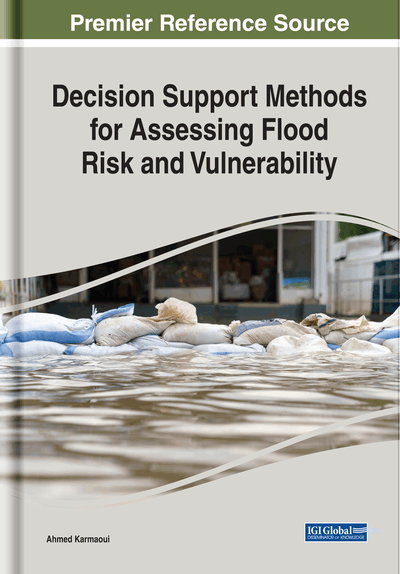# Flood Frequency Analysis Using Bayesian Paradigm: A Case Study From Pakistan

Ishfaq Ahmad (International Islamic University, Pakistan), Alam Zeb Khan (International Islamic University, Pakistan), Mirza Barjees Baig (King Saud University, Saudi Arabia) and Ibrahim M. Almanjahie (King Khalid University, Saudi Arabia)
DOI: 10.4018/978-1-5225-9771-1.ch005
Available
\$37.50
No Current Special Offers

## Abstract

At-site flood frequency analysis (FFA) of extreme hydrological events under Bayesian paradigm has been carried out and compared with frequentist paradigm of maximum likelihood estimation (MLE). The main objective of this chapter is to identify the best approach between Bayesian and frequentist one for at-site FFA. As a case study, the data of only two stations were used, Kotri and Rasul, and Bayesian and MLE approaches were implemented. Most commonly used tests were applied for checking initial assumptions. Goodness of fit (GOF) tests were used to identify the best model, which indicated that the generalized extreme value (GEV) distribution appeared to be best fitted for both stations. Under Bayesian paradigm, quantile estimates are constructed using Markov Chain Monte Carlo (MCMC) simulation method for their respective returned periods and non-exceedance probabilities. For MCMC simulations, as compared to other sampler, the M-H sampling technique was used to generate a large number of parameters. The analysis indicated that the standard errors of the parameters' estimates and ultimately the quantiles' estimates using Bayesian methods remained less as compared to maximum likelihood estimation (MLE), which shows the superiority of Bayesian methods over conventional ones in this study. Further, the safety amendments under two techniques were also calculated, which also show the robustness of Bayesian method over MLE. The outcomes of these analyses can be used in the selection of better design criteria for water resources management, particularly in flood mitigation.
Chapter Preview
Top

## Introduction

Hydrologists, over the years, have preferred to use best estimation methods and the most suitable probability distribution for extreme events through at-site FFA (Ahmad et al., 2015). Selection of suitable probability distribution not only provides the best fit to the selected site(Ahmad et al., 2013), but also provides efficient and accurate quantiles estimates corresponding to different returned periods (Ahmad et al., 2016). After finding the most suitable distribution for extreme events in FFA the next step is parameters estimation. Different approaches are utilized to estimate parameters of the respective distribution such as Method of Moments (MOM), MLE, and Method of Linear Moments (MLM). Exception of these methods, Bayesian approach based on the theorem by Thomas Bayes in 1763 (Keynes, (1921), is also intensively studied and applied now days in FFA(Coles et al.,1996) and (Gelman et al.,1997). One of the major differences between traditional approaches and Bayesian approach is that the parameters of interest have their own probability distributions and are treated as random variable rather than fixed as in traditional methods. In Bayesian approach, the sample information and prior knowledge are combined to get the posterior knowledge that provides a hypothetically consistent structure of hydrological information in the estimation of uncertainties and flood frequency models. It allows the explicit uncertainties due to flood frequency model and its parameters (Vicens et al., 1975) and (ZhongMin et al., 2011). In this context, Wood and Rodriguez-Iturbe evolved the procedures useful for dealing with the uncertainties resulting from flood frequency competing models and their parameters estimation and also compared these results with Bayesian paradigm (Wood and Rodriguez, 1975). Tang practiced a Bayesian regression analysis to estimate the resulting probability distribution of flood level (Tang et al., 1980). Van Gelder et al. the extension to Tang’s work, adopting the procedure of Bayes factor in selection of suitable probability distribution and declared that this procedure provides better results than Tang’s work (Van et al., 1999). Kuczera introduced the empirical Bayes technique to deduce hydrological quantities by joining site-specific as well as regional information. A Monte Carlo Bayesian procedure for finding the expected probability distribution with quantile confidence intervals (Kuczera, 1982) and (Kuczera, 1999). O’Connell et al. presented a comprehensive Bayesian approach to integrate historical information as well as measurement errors in the flood frequency analysis (O'Connell et al., 2002). Reis et al. established a Bayesian methodology for the analysis of a generalized least squares (GLS) regression model for regional analyses of hydrological data (Reis and Stedinger, 2005). Reis and Stedinger presented the Bayesian MCMC method for finding the posterior distributions (Reis et al., 2005). Seidou et al. offered another Bayesian method for merging local and regional knowledge to present the probability distribution of parameters (Seidou et al., 2006). Ribatet et al. presents a regional Bayesian Point Over Threshold (POT) model for FFA in the absence of at-site stream flows (Ribatet et al., 2007). More recently, Micevski and Kuczera offered a Bayesian approach for the GLS regional regression model, which could be utilized with any available at-site information to get design floods estimates with high accuracy (Micevski and Kuczera, 2009). The main purpose of Bayesian analysis in statistical hydrology is to acquire an accurate future prediction of extreme events. These results are helpful to make the design structure in hydrology. In this study, Bayesian MCMC method is utilized for estimation of the posterior distributions. Bayesian MCMC approach offers a computationally and conceptually simple way of suitably integrate (FFA) in the joint distribution of possible errors within rating curves and individual observations (Kuczera, 1999) and (Reis and Stedinger, 2005). Dissimilar sampling approaches may direct to different MCMC algorithms, which is extensively used and applied in hydrological flood frequency analysis. There are many ways to creating these chains; however Metropolish-Hastings algorithm is used in this paper (Metropolis et al., 1953).In general, the basic aim of analysis for flood frequency in extreme hydrology is to estimate the expected values of the extreme flood flows in the future i.e. the future return level or quantile estimates. Bayesian analysis is preferable due to the prediction of return level which is based on quantile estimates can be estimated easily.

## Complete Chapter List

Search this Book:
Reset